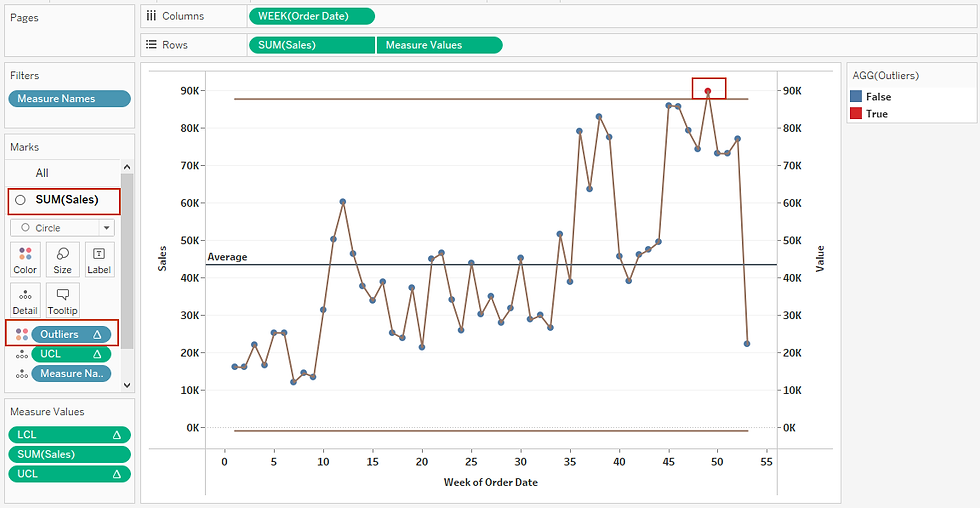top of page
Search
• Bernard K

# How to create control charts in Tableau

Updated: Jun 30, 2021### Definition

Control chart is a statistical tool used to determine if a manufacturing or business process is in a state of control. Control chart uses standard deviations above and below the mean, which are displayed as bands - with outliers identified using color.

Standard deviation is used to show how far a way data is from the mean. In this chart, the measure of standard deviation is used to identify outliers in the data. (Data points that differ significantly from other observations).

A control chart consists of:

• Points representing measurements in samples taken from a process at different times.

• The mean of all measurements in the sample.

• A center line is drawn at the mean.

• The control limits – UCL – the upper control limit and LCL – the lower control limit.

• Signals – Outliers (any point outside the control limits)

### Example of a control chart### How to create a control chart in Tableau

In this article, using Sample-Superstore data I am going to show you two ways you can create a control chart in Tableau.

### Option one

Create a simple timeline by dragging WEEK(Order Date) to the columns and SUM(Sales) to the rows shelf.

Choose Line chart under marks card.Right-click on the y-axis and add a reference line showing the average value.Next, right click on the y-axis and add a reference distribution of +2 and -2 standard deviation.

Alternatively, you can also use +3 and -3 or +1 and -1 standard deviation.Executing these results to.### Option two

Create a simple timeline again by dragging WEEK (Order Date) to the columns and SUM(Sales) to the rows shelf.

Choose circle under marks card.Create UCL (upper control limit) calculation as follows.(Note, this calculation computes UCL at 2 standard deviations from the mean. You can change this to 3 standard deviations by simply replacing 2 with 3 in the calculation or use a parameter to empower users choose the number of standard deviations)

Create LCL (lower control limit) calculation as follows.Next, drag measure values to the rows shelf and eliminate all measures from the view except, LCL, UCL and SUM(Sales).

Change the marks card of the second view to a line chart.Make chart dual axis and synchronize the axis.Next, right-click on the y-axis and add a reference line showing the average value – just like in the first option of creating a control chart.Next, lets color the circles – so to empower users easily spot outliers using the following calculation.Drag the above calculation to the color shelf of the first view.(Where you can see that there is one point that falls outside the control limit (one outlier)).

### Let’s parameterize the control chart.

Let’s make the control chart dynamic by empowering users choose how many standard deviations to be used in computing the upper and lower control limits.

Create a parameter.Replace the 2 on the UCL and LCL calculations with this parameter – ‘Standard deviation’.Show the parameter control.

Note, now you can easily recomputed the control limits by selecting the number of standard deviations to be used – on the parameter control.